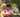### Brackets

January 31, 2020

Brackets

A string S consisting of N characters is considered to be properly nested if any of the following conditions is true:
• S is empty;
• S has the form "(U)" or "[U]" or "{U}" where U is a properly nested string;
• S has the form "VW" where V and W are properly nested strings.

For example, the string "{[()()]}" is properly nested but "([)()]" is not.

Write a function:
function solution(S);
that, given a string S consisting of N characters, returns 1 if S is properly nested and 0 otherwise.

For example, given S = "{[()()]}", the function should return 1 and given S = "([)()]", the function should return 0, as explained above.

Write an efficient algorithm for the following assumptions:
• N is an integer within the range [0..200,000];
• string S consists only of the following characters: "(", "{", "[", "]", "}" and/or ")".

Hints
• push left brackets into an array L1
• compare non-left brackets with L1.pop()

``````function isPair(c1, c2){
if( c1=='(' && c2==')' ) return true
if( c1=='{' && c2=='}' ) return true
if( c1=='[' && c2==']' ) return true
return false
}

function solution(S) {
// write your code in JavaScript (Node.js 8.9.4)
if(S.length < 1) return 1

let L = ['(', '{', '[']
let L1 = []
let c

for(let i=0; i<S.length; i++){
if( L.includes(S[i])){ // Left brackets
L1.push(S[i])
} else { // Right brackets
if(L1.length < 1) return 0 // no left barckets for matching
c = L1.pop()
if( !isPair(c, S[i])) return 0
}
}
if( L1.length < 1) return 1 // all popped out
else return 0 // Let brackets remaining
}``````Posted by Tai Lu ( 呂台生 ) who's building useful things.You should follow him on Twitter